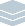Wolfram Archive

# The Ease of Wolfram|Alpha, the Power of Mathematica: Introducing Wolfram|Alpha Notebook Edition

Published September 12, 2019

Wolfram|Alpha has been a huge hit with students. Whether in college or high school, Wolfram|Alpha has become a ubiquitous way for students to get answers. But it’s a one-shot process: a student enters the question they want to ask (say in math) and Wolfram|Alpha gives them the (usually richly contextualized) answer. It’s incredibly useful—especially when coupled with its step-by-step solution capabilities. But what if one doesn’t want just a one-shot answer? What if one wants to build up (or work through) a whole computation?

Well, that’s what we created Mathematica and its whole notebook interface to do. And for more than 30 years that’s how countless inventions and discoveries have been made around the world. It’s also how generations of higher-level students have been taught.

But what about students who aren’t ready to use Mathematica yet? What if we could take the power of Mathematica (and what’s now the Wolfram Language), but combine it with the ease of Wolfram|Alpha?

Well, that’s what we’ve done in Wolfram|Alpha Notebook Edition.